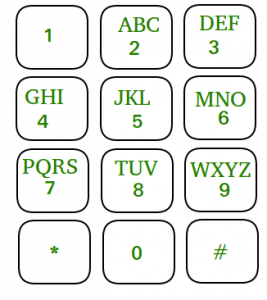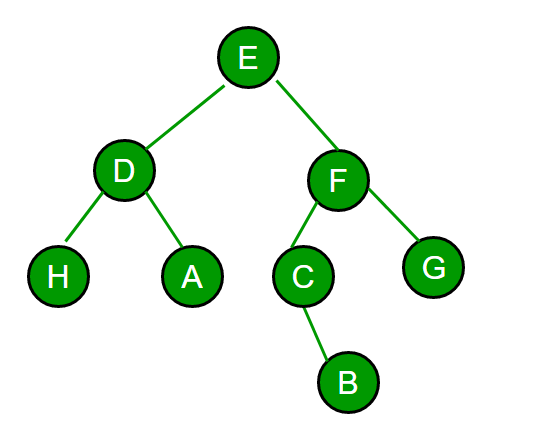# Flipkart Interview | Set 11

Checking your coding capabilities and thinking level is their main motive. Hence the whole procedure I was involved in dealt only with coding questions. 😀

There were total 4 Rounds.

1. Online Coding
2. Machine Coding
3. Face to face Technical Interview
4. Technical + HR
• Round 1 : Online Coding :

Time: 1 hour 30 Min

1. Powers of 5
Cutting Binary String

2. Apllication of DFS/BFS
You were given a directed graph with n nodes. Given graph was connected. If there is an edge from u to v then u depends on v. Our task was to find out the sum of dependencies for every node.

For the graph in diagram, A dependencies: C,D i.e. 2
B dependencies: D i.e. 1
C dependencies: D i.e. 1
And D depends on none.

• Round 2: Machine Coding Round

Time: 1 hour 30 min

1. Given two very large numbers a & b in string format. You need to produce the result for the following operation:
1: Subtract a & b
2: Multiply a & b

Example:
123456789
987654321

Output:
0 → 1111111110
1 → -864197532
2 → 1219326313718945259

Few cases were checked specifically:

• Output of 132-132 must be 0 not 000.
• 2 – 122222000002 should produce -122222000000 i.e either a or b can be the bigger string.
• Negative input should be handled. Result of -2+202 = 200.
• 1000000000 – 1 = 999999999 i.e. resulting answer can be of different length.

• Round 3: Face to face technical Interview

Time: 45-50 minutes.

• Given the mobile numeric keypad. You can only press buttons that are up,left,right or down to the current button.You are not allowed to press bottom row corner buttons (i.e. * and # ).Given a N find out the number of numbers possible of given length. It was asked to write the code.

Example:
For N=2
Possible numbers: 00,08 11,12,14 22,21,23,25 and so on. We have to print the count of such numbers.
I solved it using DP in the first attempt. He was quite impressed as I took very less time to think of the exact solution.

• Given an undirected graph with the following special points:
1. Every node has at most 3 links.
2. Node with a single link is a leaf node.
3. Nodes are numbered from 1 to N.

(Think how is it different from a 3-ary Tree, See bold text 😛 )
We do the following operation on the given graph: Among all the current leaf nodes we search for the node with minimum node value, delete it and print its parent. We do this operation until only two nodes are left in the graph.
If you are given N and an array having the printed values from the operation, you have to generate the graph back. Exact implementation was asked to write.

Example:
N = 8

ar[]= {4, 3, 6, 6, 5, 4}

Output should be adjacency matrix for the following graph.Example Image for Question 2 of Round 3.

• Round 4 : Technical + HR

Time: 1 Hour

1. Introduction.
2. Asked everything about my project every minute detail, even I was asked to write code for a few sections of my code i.e. how I implemented those features.
3. Asked few questions on teamwork that how would I handle particular situations.
4. Strengths and weaknesses.
5. Tell me about one thing that you have done in college and proud of being it in academics or in any field.
6. Best and worst classmate till now and Why !! 😀

With this as the final interview, my long journey of Interviews ended :). Wish you all, all the very best hope this would help 🙂

• Following are round 1 online coding questions.

1. Summing Nodes (Programming)
At Flipkart, an employee has many subordinates. But an employee can also have many managers. And a manager can further have more managers overseeing his work.

A simple “boss-employee-relationship” can be denoted by an arrow ‘—>’
A → B → C
This denotes that A is the direct boss of B, who is the direct boss of C. Here A is also the (indirect) boss of C. It is guaranteed that there is no cycles in this relationship.
That is there exists no pair of employees, X and Y, such that X is boss of Y and Y is also boss of X.

The salary of an employee can be calculated on the basis of the following rules:

1. an employee like C who has no subordinates earns 1.
2. An employee who has direct subordinates earns a salary equal to the sum of his direct subordinates’ salaries.

“relations” denotes an array of strings, where if the ith character of ith string is Y, if employee i is a direct boss of employee j. Otherwise, it is ‘N’. You have to display the sum of salaries of all employees.

Complete this function in the code editor:
int Summing(String[] relations)

Constraints

• The size of relations array will not have elements greater than 50.
• the arrays will only contain string composed of ‘Y’ & ‘N’.
• each element of the array has the same number of characters.
• For kth row, the kth element will always be ‘N’.
• If X is boss of Y, Y cannot be a boss of X.
• Test cases are designed such that answers will always lie within the range of a signed 32-bit int.

Sample Case #1
Input
N
Returns: 1
Explanation: There is only one employee, so his salary will be 1.

Sample Case #2
Input
NNYN
NNYN
NNNN
NYYN
Returns: 5
Explanation: It has the following relation.

 `   ``4 ` `   ``| \ ` `1  |  2 ` ` ``\ | /  ` `   ``3 `

So the salary of 3rd employee is 1, 1st and 2nd employee is equal to the sum of its (only) employee (3rd) which is 1. Salary of 4th employee is the sum of the salary of 2nd and 3rd employee which is 1 + 1 = 2. So total salary is 2 + 1 + 1 + 1 = 5.

2. The future is not always what we expect it to be. 60 years ago, we never thought that the world of humans, as we know it, will undergo such a drastic change. In the final war between the humans and the apes, the apes won, and Ceaser leads his civilization to its zenith,” a fellow human prisoner tells another.

“Now some of them have even learnt mathematics and are trying their best to re-create human machines from ruins. They have asked me to help them start a power generator, or else they’ll have no reason to keep me alive.”

To start it, a puzzle has to be solved:
You have to complete a function int optimalCuts(string s), where s is a string containing 0’s and l’s. It has to return the smallest positive integer C, such that, the bit string can be cut into C pieces and each piece is a power of 5. Note that each piece can also represent different power of 5. If no such cut is possible then return -1.

Constraints

1. Argument (Strings) s) will consists of only ‘0’ and ‘1’.
2. 1 <= length(s) <= 50.

Sample Case #1
s = 101101101
Returns: 3
Explanation: We can split the given string into three “101”s, where 101 is the binary representation of 5.

Sample Case #2
s = 1111101
Returns: 1
Explanation: “1111101” is 125 which is 5^3

Sample Case #3
s = 00000
Returns: -1
Explanation: 0 is not a power of 5.

If you like GeeksforGeeks and would like to contribute, you can also write an article and mail your article to contribute@geeksforgeeks.org. See your article appearing on the GeeksforGeeks main page and help other Geeks.

Write your Interview Experience or mail it to contribute@geeksforgeeks.org

My Personal Notes arrow_drop_up